# AP Chemistry : Integrated Rate Laws

## Example Questions

### Example Question #1 : Integrated Rate Laws

The rate constant for a second-order reaction is 0.15 M-1s-1.  If the initial concentration of the reactant is 0.30 M, how long does it take for the concentration to decrease to 0.15 M?

253 second

22.2 seconds

11.1 seconds

88.8 seconds

44.4 second

22.2 seconds

Explanation: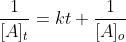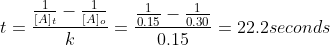### Example Question #1 : Integrated Rate Laws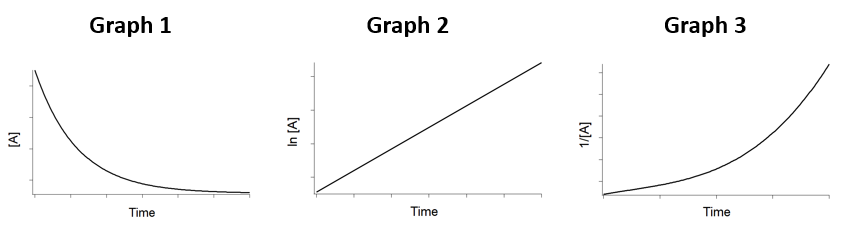Based on the figure above, what is the order of reaction?

Third Order

Second Order

Zero Order

First order

Squared Order

First order

Explanation:

For a first order reaction, the ln [A]t is linear with t.

### Example Question #1 : Integrated Rate Laws

A compound decomposes by a first-order process.  If 25.0% of the compound decomposes in 60 minutes, the half-life of the compound is?

65 minutes

180 minutes

198 minutes

120 minutes

145 minutes

145 minutes

Explanation: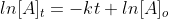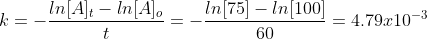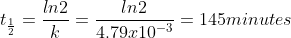### Example Question #41 : Kinetics And Energy

Cyclopentane is unstable and decomposes by a first order reaction.  The rate constant for this reaction is 9.5 s-1.  What is the half life of the reaction?

0.132 seconds

0.0314 seconds

0.0729 second

0.0582 seconds

0.0614 seconds

0.0729 second

Explanation: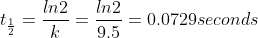### Example Question #1 : Integrated Rate Laws

The half life of a first order reaction is 1.5 hours.  What is the rate constant of this reaction?

1.5

0.75

0.52

0.21

0.46

0.46

Explanation: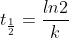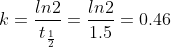### All AP Chemistry Resources